Next: Bibliography Up: tr01cb1 Previous: Acknowledgements

# Appendix

The general form of the Sherman-Morrison-Woodbury formula  is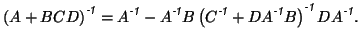(18)

Also, the inverse for a matrix in block form is given by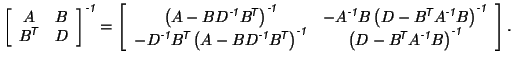Theorem A:

Any model of the form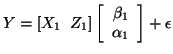can be rewritten as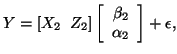where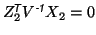whilst being completely equivalent in terms of the estimated parameters of interest (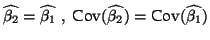) and the modelled signal space: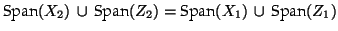in the pre-whitened space. Note that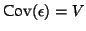for both models.

That is, the signals of interest can be made orthogonal to the confounds without affecting the estimation of the parameters or the residuals.

Proof:

The proof is by construction, where we show that orthogonalising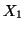with respect to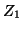gives the desired results. Let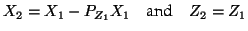where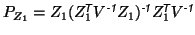is the projection matrix forin the pre-whitened space.

These equations give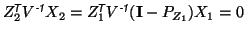. Also, the combined span of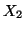and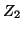is clearly the same as that ofand.

Now consider the covariances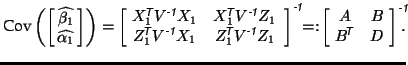Using the block matrix inverse, this gives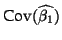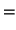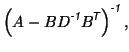while, since the off-diagonal blocks are zero in the second case, the calculation simply gives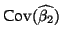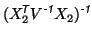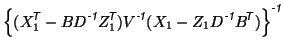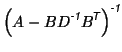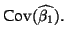For the first model, the parameter estimates, given by equation 5, can be written using the matrix block inversion formula, giving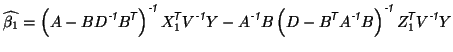(19)

while for the second model, the block diagonal form yields the familiar form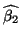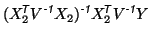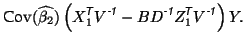Applying the Sherman-Morrison-Woodbury formula to the second term in equation 12 gives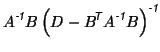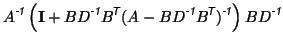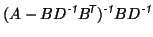Substituting this into equation 12 gives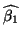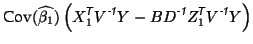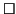Theorem B:

Given the standard GLM,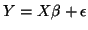, and a set of linearly independent contrasts specified by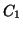such that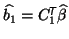, then an equivalent model without contrasts, but with confounds, exists in the form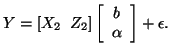That is,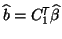,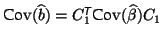and the modelled signal space: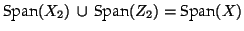in the pre-whitened space. Note thatfor both models.

Proof:

The proof is, again, by construction. Firstly, let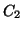be a set of contrasts that when combined withform a complete linearly independent set of contrasts. That is, the matrix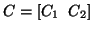will be full rank (and hence invertible). Then let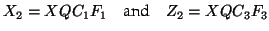where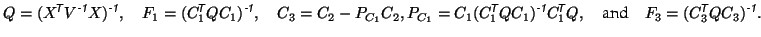From these definitions it is easy to see that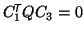, which represents an orthogonality condition. As before, it is straightforward to verify that the combined span ofandis equal to the span of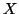. Consequently,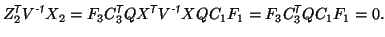Therefore,andare orthogonal as well.

The estimation equations for the model become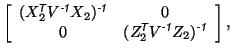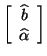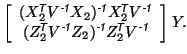Thus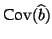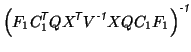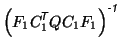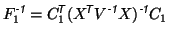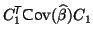and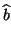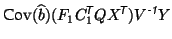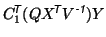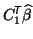Next: Bibliography Up: tr01cb1 Previous: Acknowledgements
Christian Beckmann 2003-07-16